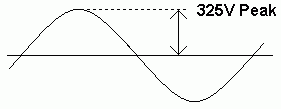RANDOM PAGE

SITE SEARCH

LOG
IN

HELP

# Root Mean Square (RMS)

This is the AQA version closing after June 2019. Visit the the version for Eduqas instead.

## Root Mean Square

In AC circuits, measurements are usually in RMS instead of Peak.

### RMS Volts and Amps

230 Volts RMS Alternating Current (AC) has the same heating effect as 230 Volts Direct Current (DC).

RMS = Root Mean Square

```Vrms = 0.7 Vpeak

Vpeak = 1.4 Vrms
```

Alternating voltages (sine waves) have an average voltage of zero because they spend equal times above and below the zero volt line. Clearly it is not useful to say the AC mains supply voltage is zero. The RMS calculation works like this.

• Square: The voltage is squared. This makes any negative parts positive
• Root: The voltage is square rooted to undo the squaring but - the negative parts are still positive
• Mean: Take the average

The mains supply in Europe is 230 Volts RMS. The peak voltage is 325 Volts.0.707 is approximately equal to 0.7 = 1 / sqrt(2)

### RMS Power

```Prms = 0.5 Ppeak

Ppeak = 2 Prms
```

reviseOmatic V3     Contacts, ©, Cookies, Data Protection and Disclaimers Hosted at linode.com, London